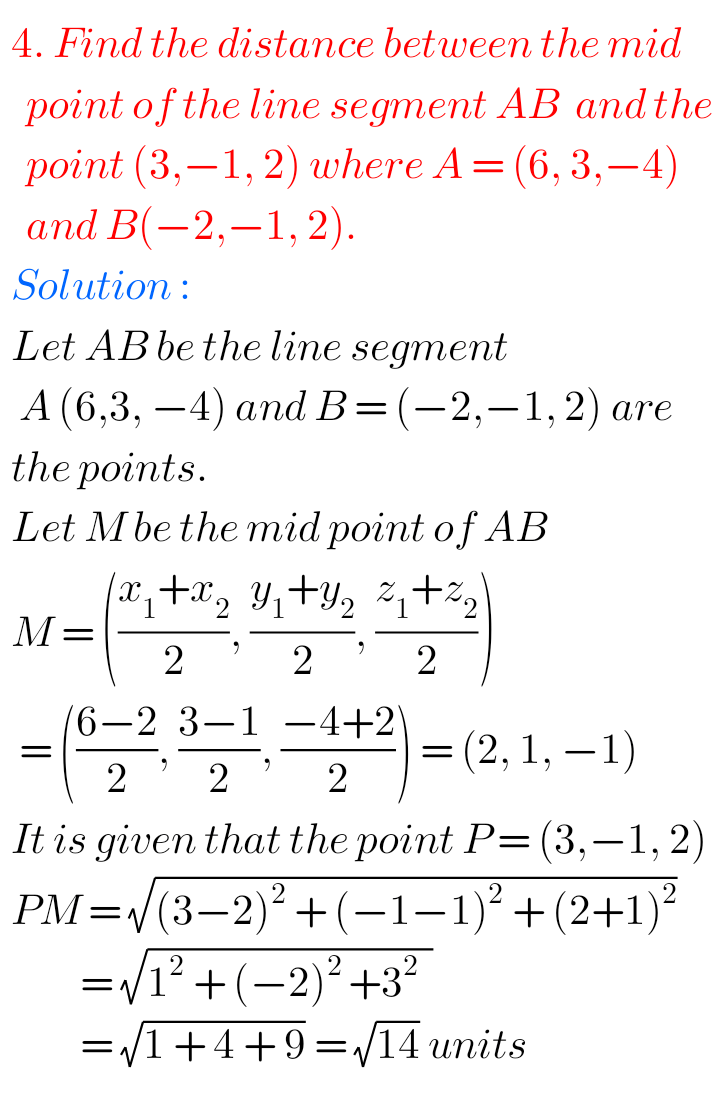# Maths 1b Chapter 5 Inter solutions.## Inter maths solutions for Three Dimensional Coordinates exercise 5(b)

Three Dimensional Coordinates solutions Inter exercise 5(b) Intermeduate Mathematics 1B Chapter 5 Three Dimensional Coordinates Exercise 5(b) textbook solutions are given. First you sytudy the textbook lesson very well. Then observe the example problems and solutions given in the textbook. The keypoints in the lesson are very important. Observe the solutions given below and try …## Inter Maths 1B solutions for Three Dimensional Coordinates Exercise 5(a)

Three Dimensional Coordinates solutions Inter Intermeduate Mathematics 1B Chapter 5 Three Dimensional Coordinates Exercise 5(a) textbook solutions are given. First you sytudy the textbook lesson very well. Then observe the example problems and solutions given in the textbook. The keypoints in the lesson are very important. Observe the solutions given below and try them in …